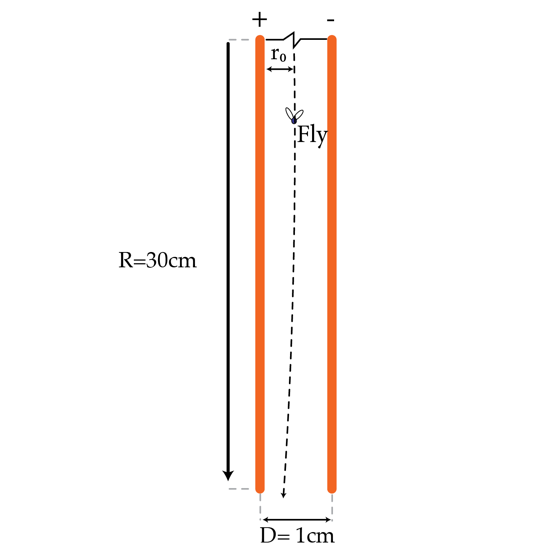# The flight of the housefly

A housefly is sitting between the plates of a parallel-plate capacitor. The plates of the capacitor are disks of radius $R=30 cm$ separated by a distance $d=1 cm$. The distance between the fly and the positive plate of the capacitor is $r_{0}=\frac{d}{2}(1-\epsilon)$ with $\epsilon=10^{-4}$ ( note that the fly is very close to the center of the capacitor). The housefly decides to exit the capacitor by flying along an equipotential line. What is the maximum distance in meters from the center of the capacitor the housefly reaches along the equipotential line?×

Problem Loading...

Note Loading...

Set Loading...